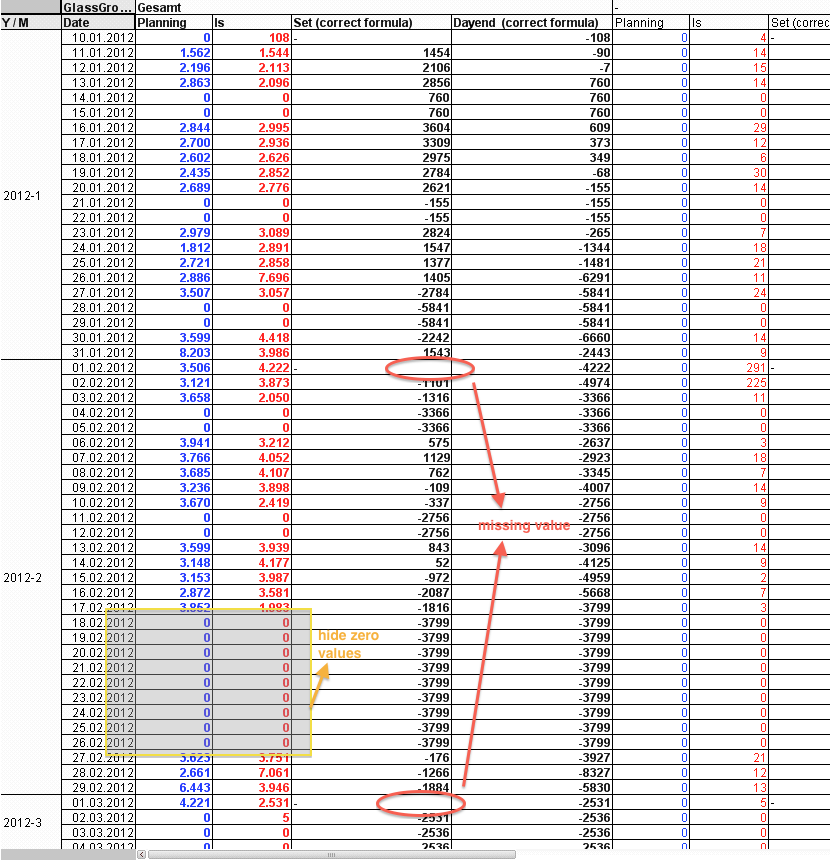# QlikView Layout & Visualizations

Discussion Board for collaboration on QlikView Layout & Visualizations.

HighlightedNot applicable

## Column reference calculation in pivot tables as Excel, help!

Hi!

Can someone help me with the following problem.
I have a pivot table where the formula needs to be adjusted.
The formula referenced on calculated columns.
How do I get the formula correctly adjusted so that the result corresponds to the attached Excel (column Set and the Dayend - correct formula).

Furthermore, the calculation for the column Dayend should be stored when I select the month 2 in the Listbox.

For example when I select the second month then the Dayend is to be taken from the first month -> last day from first month

(Dayend before = -2443 for the Day 31.01.2012).

Thanks for your help.

Screenshot from Excel:5 Replies
Contributor III

## Re: Column reference calculation in pivot tables as Excel, help!

See the attached file.  I've added two new expresisons.  The labels are in bold.

Set (correct formula)

Set (correct formula) - Is

Dayend  (correct formula)

if(isnull([Set (correct formula)]),0,[Set (correct formula)]) - IsNot applicable

## Re: Column reference calculation in pivot tables as Excel, help!

Hi Mark!

Thank you for your help that ist what I need, but I miss values on the month break!. How I can get this values?

Is it possible to hide the zero values ​​of the rows when I use the inter record function (above etc...)

See attached screenshot.

Thank you!Contributor III

## Re: Column reference calculation in pivot tables as Excel, help!

See the attached file, not exactly straghtforards and probaly would have been better to do this in the script...

MarkNot applicable

## Re: Column reference calculation in pivot tables as Excel, help!

Hi Mark!

This is a great solution, but when I select the 2nd month then the set and dayend column loses the previously calculated values.

This means the dayend is based on a different value then before.

See attached file.

How can I aggregate these values ​​so that they do not get lost if you select something!

ThanksContributor III

## Re: Column reference calculation in pivot tables as Excel, help!

To do this you'll need to use set analysis and remove the selections you don't want (probably all of your dimensions)

sum({\$<Ma_Networkdays=,GL\$SYS_Gruppe=,Ma_Jahr_Monat=,Ma_Networkdays = Datum_GLSTRT>} GLPQOR/1000)

The problem is you can't do the equivilent of "Ma_Networkdays = Datum_GLSTRT" in set analysis to reduce the data set.  I don't understand your data model but you really should avoid having to do such expressions in the script.